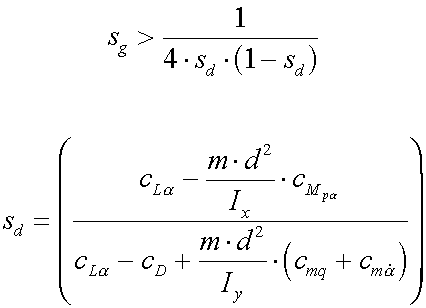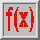# The dynamic stability condition## Abbreviations

 cD Drag coefficient cLa Lift coefficient derivative cMpa Magnus moment coefficient derivative cmq+cma Pitch damping moment derivative sg Gyroscopic (static) stability factor sd Dynamic stability factor

## Explanation

A projectile is said to be dynamically stable, if its yawing motion of nutation and precession is damped out with time, which means that an angle of yaw induced at the muzzle (the initial yaw) decreases.

A dynamic stability factor sd can be defined from the linearized theory of gyroscopes (assuming only a small angle of yaw) and the above dynamic stability condition can be formulated. An alternate formulation of this conditionleads to the illustrative stability triangle.

sd however depends on five aerodynamic coefficients. Because these coefficients are hard to determine, it can become very complicated to calculate the dynamic stability factor, which varies as a function of the momentary bullet velocity.